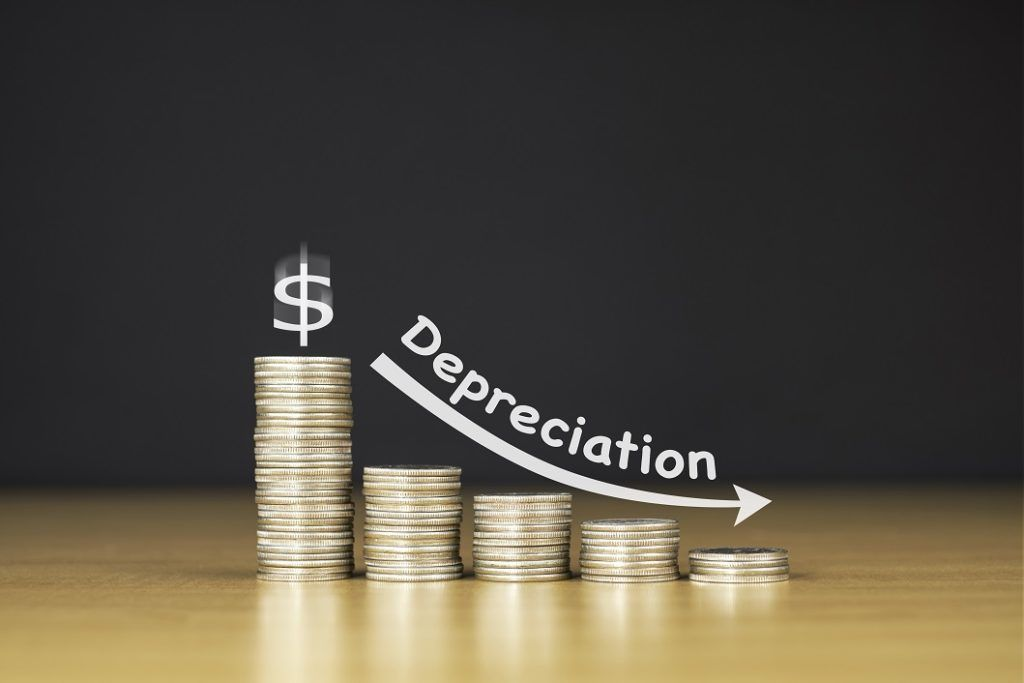Economic Depreciation

The decline in the economic value of an asset over time

What is Economic Depreciation?

Economic depreciation is the decline in the economic value of an asset over time. It is also known as consumption of fixed capital for the purpose of estimating national accounts. Economic depreciation can be attributed to indirect factors such as a decline in the quality of living, deterioration in the quality of neighborhood roads, etc.Depreciation and the Capital Accumulation Equation

The capital accumulation equation links present capital stock to future capital stock. The capital accumulation equation considers either proportional depreciation (i.e., a constant fraction of the existing capital stock is lost to depreciation) or constant depreciation (i.e., a constant amount of the existing capital stock is lost to depreciation).

Proportional Depreciation: Kt+1 = (1 – δ)Kt + It

Constant Depreciation: Kt+1 = Kt – δ + It

Where:

• Kt – the capital stock level at time period t (the current time period)
• Kt+1 – the capital stock level at time period t+1 (the future time period)
• It – the investment made in capital stock at time period t
• δ – the coefficient of depreciation

Causes of Economic Depreciation

1. Wear and tear

Wear and tear is inevitable over time. The decline in the physical value of an asset is accounted for by depreciation by depreciating the financial value of the asset.

2. New technology replacing old technology

Because rapid technological changes come into place every few years, existing technology becomes outdated and is replaced by more efficient technology. It leads to depreciation in the value of the outdated asset.

3. Perishability

Certain assets, like work in progress assets such as raw materials or inventory, etc. deteriorate over a quick span of time. Such assets are subject to depreciation to account for the rapid loss in their value.

4. Expiration of rights

Intangible assets such as software, license, patents, trademarks, etc. are valid through a span of time, the amount of time for which the rights for the same are contracted for. Intangible assets need to be depreciated before the rights expire. Depreciation for intangible assets is called amortization, and intangibles are amortized over time such that when the rights expire, the value of the intangibles is usually zero.

More Resources

CFI is the official provider of the global Financial Modeling & Valuation Analyst (FMVA)™ certification program, designed to help anyone become a world-class financial analyst. To keep advancing your career, the additional resources below will be useful:

• Accounting Profit vs Economic Profit
• Consumption
• Depreciation Methods
• Keynesian Economic Theory

Financial Analyst Certification

Become a certified Financial Modeling and Valuation Analyst (FMVA)® by completing CFI’s online financial modeling classes!